Previous Page | Next Page

 The GLIMMIX Procedure

## Example 38.11 Maximum Likelihood in Proportional Odds Model with Random Effects

The data for this example are taken from Gilmour, Anderson, and Rae (1987) and concern the foot shape of 2,513 lambs that represent 34 sires. The foot shape of the animals was scored in three ordered categories. The following DATA step lists the data in multivariate form, where each observation corresponds to a sire and contains the outcomes for the three response categories in the variables k1, k2, and k3. For example, for the first sire the first foot shape category was observed for 52 of its offspring, foot shape category 2 was observed for 25 lambs, and none of its offspring was rated in foot shape category 3. The variables yr, b1, b2, and b3 represent contrasts of fixed effects.

```data foot_mv;
input yr b1 b2 b3 k1 k2 k3;
sire = _n_;
datalines;
1  1  0  0  52 25  0
1  1  0  0  49 17  1
1  1  0  0  50 13  1
1  1  0  0  42  9  0
1  1  0  0  74 15  0
1  1  0  0  54  8  0
1  1  0  0  96 12  0
1 -1  1  0  57 52  9
1 -1  1  0  55 27  5
1 -1  1  0  70 36  4
1 -1  1  0  70 37  3
1 -1  1  0  82 21  1
1 -1  1  0  75 19  0
1 -1 -1  0  17 12 10
1 -1 -1  0  13 23  3
1 -1 -1  0  21 17  3
-1  0  0  1  37 41 23
-1  0  0  1  47 24 12
-1  0  0  1  46 25  9
-1  0  0  1  79 32 11
-1  0  0  1  50 23  5
-1  0  0  1  63 18  8
-1  0  0 -1  30 20  9
-1  0  0 -1  31 33  3
-1  0  0 -1  28 18  4
-1  0  0 -1  42 27  4
-1  0  0 -1  35 22  2
-1  0  0 -1  33 18  3
-1  0  0 -1  35 17  4
-1  0  0 -1  26 13  2
-1  0  0 -1  37 15  2
-1  0  0 -1  36 14  1
-1  0  0 -1  63 20  3
-1  0  0 -1  41  8  1
;
```

In order to analyze these data as multinomial data with PROC GLIMMIX, the data need to be arranged in univariate form. The following DATA step creates three observations from each record in data set foot_mv and stores the category counts in the variable count:

```data footshape; set foot_mv;
array k{3};
do Shape = 1 to 3;
count = k{Shape};
output;
end;
drop k:;
run;
```

Because the sires were selected at random, we consider here a model for the three-category response with fixed regression effects for yr, b1b3, and with random sire effects. Because the response categories are ordered, a proportional odds model is chosen (McCullagh 1980). Gilmour, Anderson, and Rae (1987) consider various analyses for these data. The following GLIMMIX statements fit a model with probit link for the cumulative probabilities by maximum likelihood where the marginal log likelihood is approximated by adaptive quadrature:

```proc glimmix data=footshape method=quad;
class sire;
model Shape = yr b1 b2 b3 / s link=cumprobit dist=multinomial;
random int / sub=sire s cl;
ods output Solutionr=solr;
freq count;
run;
```

The number of observations that share a particular response and covariate pattern (variable count) is used in the FREQ statement. The S and CL options request solutions for the sire effects. These are output to the data set solr for plotting.

The "Model Information" table shows that the parameters are estimated by maximum likelihood and that the marginal likelihood is approximated by Gauss-Hermite quadrature (Output 38.11.1).

Output 38.11.1 Model and Data Information
The GLIMMIX Procedure

Model Information
Data Set WORK.FOOTSHAPE
Response Variable Shape
Response Distribution Multinomial (ordered)
Variance Function Default
Frequency Variable count
Variance Matrix Blocked By sire
Estimation Technique Maximum Likelihood
Degrees of Freedom Method Containment

 Number of Observations Read 102 96 2513 2513

Response Profile
Ordered
Value
Shape Total
Frequency
1 1 1636
2 2 731
3 3 146

The distribution of the data is multinomial with ordered categories. The ordering is implied by the choice of a link function for the cumulative probabilities. Because a frequency variable is specified, the number of observations as well as the number of frequencies is displayed. Observations with zero frequency—that is, foot shape categories that were not observed for a particular sire are not used in the analysis. The "Response Profile Table" shows the ordering of the response variable and gives a breakdown of the frequencies by category.

Output 38.11.2 Information about the Size of the Optimization Problem
Dimensions
G-side Cov. Parameters 1
Columns in X 6
Columns in Z per Subject 1
Subjects (Blocks in V) 34
Max Obs per Subject 3

Optimization Information
Optimization Technique Dual Quasi-Newton
Parameters in Optimization 7
Lower Boundaries 1
Upper Boundaries 0
Fixed Effects Not Profiled
Starting From GLM estimates

With METHOD=QUAD, the "Dimensions" and "Optimization Information" tables are particularly important, because for this estimation methods both fixed effects and covariance parameters participate in the optimization (Output 38.11.2). For GLM models the optimization involves the fixed effects and possibly a single scale parameter. For mixed models the fixed effects are typically profiled from the optimization. Laplace and quadrature estimations are exceptions to these rules. Consequently, there are seven parameters in this optimization, corresponding to six fixed effects and one variance component. The variance component has a lower bound of 0. Also, because the fixed effects are part of the optimizations, PROC GLIMMIX initially performs a few GLM iterations to obtain starting values for the fixed effects. You can control the number of initial iterations with the INITITER= option in the PROC GLIMMIX statement.

The last entry in the "Optimization Information" table shows that—at the starting values—PROC GLIMMIX determined that a single quadrature point is sufficient to approximate the marginal log likelihood with the required accuracy. This approximation is thus identical to the Laplace method that is available with METHOD=LAPLACE.

For METHOD=LAPLACE and METHOD=QUAD, the GLIMMIX procedure produces fit statistics based on the conditional and marginal distribution (Output 38.11.3). Within the limits of the numeric likelihood approximation, the information criteria shown in the "Fit Statistics" table can be used to compare models, and the2 log likelihood can be used to compare among nested models (nested with respect to fixed effects and/or the covariance parameters).

Output 38.11.3 Marginal and Conditional Fit Statistics
Fit Statistics
-2 Log Likelihood 3870.12
AIC (smaller is better) 3884.12
AICC (smaller is better) 3884.17
BIC (smaller is better) 3894.81
CAIC (smaller is better) 3901.81
HQIC (smaller is better) 3887.76

Fit Statistics for Conditional Distribution
-2 log L(Shape | r. effects) 3807.62

The variance of the sire effect is estimated as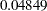with estimated asymptotic standard error of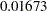(Output 38.11.4). Based on the magnitude of the estimate relative to the standard error, one might conclude that there is significant sire-to-sire variability. Because parameter estimation is based on maximum likelihood, a formal test of the hypothesis of no sire variability is possible. The category cutoffs for the cumulative probabilities are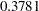and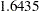. Except for b3, all fixed effects contrasts are significant.

Output 38.11.4 Parameter Estimates
Covariance Parameter Estimates
Cov Parm Subject Estimate Standard Error
Intercept sire 0.04849 0.01673

Solutions for Fixed Effects
Effect Shape Estimate Standard Error DF t Value Pr > |t|
Intercept 1 0.3781 0.04907 29 7.71 <.0001
Intercept 2 1.6435 0.05930 29 27.72 <.0001
yr   0.1422 0.04834 2478 2.94 0.0033
b1   0.3781 0.07154 2478 5.28 <.0001
b2   0.3157 0.09709 2478 3.25 0.0012
b3   -0.09887 0.06508 2478 -1.52 0.1289

A likelihood ratio test for the sire variability can be carried out by adding a COVTEST statement to the PROC GLIMMIX statements (Output 38.11.5):

```ods select FitStatistics CovParms Covtests;
class sire;
model Shape = yr b1 b2 b3 / link=cumprobit dist=multinomial;
random int / sub=sire;
covtest GLM;
freq count;
run;
```

The statement

```covtest GLM;
```

compares the fitted model to a generalized linear model for independent data by removing the sire variance component from the model. Equivalently, you can specify

```covtest 0;
```

which compares the fitted model against one where the sire variance is fixed at zero.

Output 38.11.5 Likelihood Ratio Test for Sire Variance
The GLIMMIX Procedure

Fit Statistics
-2 Log Likelihood 3870.12
AIC (smaller is better) 3884.12
AICC (smaller is better) 3884.17
BIC (smaller is better) 3894.81
CAIC (smaller is better) 3901.81
HQIC (smaller is better) 3887.76

Covariance Parameter Estimates
Cov Parm Subject Estimate Standard Error
Intercept sire 0.04849 0.01673

Tests of Covariance Parameters
Based on the Likelihood
Label DF -2 Log Like ChiSq Pr > ChiSq Note
Independence 1 3915.29 45.17 <.0001 MI

MI: P-value based on a mixture of chi-squares.

The2 Log Likelihood in the reduced model without the sire effect is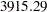. Compared to the corresponding marginal fit statistic in the full model (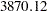), this results in a chi-square statistic of 45.17. Because the variance component for the sire effect has a natural lower bound of zero, PROC GLIMMIX performs the likelihood ratio test as a one-sided test. As indicated by the note, the p-value for this test is computed from a mixture of chi-square distributions, applying the results of Self and Liang (1987). There is significant evidence that the model without sire random effects does not fit the data as well.

In studies of heritability, one is often interested to rank individuals according to some measure of "breeding value." The following statements display the empirical Bayes estimates of the sire effects from ML estimation by quadrature along with prediction standard error bars (Output 38.11.6):

```proc sort data=solr; by Estimate;
data solr; set solr;
length sire \$2;
obs  = _n_;
sire = left(substr(Subject,6,2));
run;
proc sgplot data=solr;
scatter x=obs y=estimate /
markerchar  = sire
yerrorupper = upper
yerrorlower = lower;
xaxis grid label='Sire Rank' values=(1 5 10 15 20 25 30);
yaxis grid label='Predicted Sire Effect';
run;
```

Output 38.11.6 Ranked Predicted Sire Effects and Prediction Standard Errors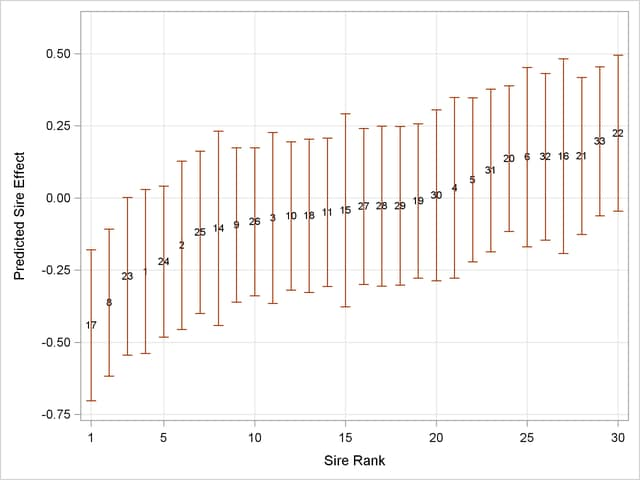Previous Page | Next Page | Top of Page